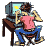Home » SQL & PL/SQL » SQL & PL/SQL » Adding Cells from two different tables (12.1.0.2)
Adding Cells from two different tables Thu, 02 June 2016 22:52bdtran Messages: 17Registered: May 2016 Junior Member
Hello,

I need a insert statement to do a calculation of adding cells from two different tables, TBSIGNAL1 and TBSIGNAL2. The result will be inserted into the TBSIGNAL3 as below
SID1C1 of TBSIGNAL3 = SID1C1 of TBSIGNAL1 + SID1C1 of TBSIGNAL2
SID1C2 of TBSIGNAL3 = SID1C1 of TBSIGNAL3 + SID1C2 of TBSIGNAL1 + SID1C2 of TBSIGNAL2
SID1C3 of TBSIGNAL3 = SID1C2 of TBSIGNAL3 + SID1C3 of TBSIGNAL1 + SID1C3 of TBSIGNAL2
SID1C4 of TBSIGNAL3 = SID1C3 of TBSIGNAL3 + SID1C4 of TBSIGNAL1 + SID1C4 of TBSIGNAL2
And so on...

Please see table data and structure below.

All help is much appreciated.

```

-- TBSIGNAL3 should have the result below.
SID	TID	C1	C2	C3	C4	C5	C6	C7	C8	C9	C10	C11	C12
1	3	4	8	12	16	20	24	28	32	36	40	44	48
2	3	10	20	30	40	50	60	70	80	90	100	110	120
3	3	16	32	48	64	80	96	112	128	144	160	176	192

> desc TBSIGNAL3;

Name Null     Type
---- -------- ------
SID  NOT NULL NUMBER
TID  NOT NULL NUMBER
C1            NUMBER
C2            NUMBER
C3            NUMBER
C4            NUMBER
C5            NUMBER
C6            NUMBER
C7            NUMBER
C8            NUMBER
C9            NUMBER
C10           NUMBER
C11           NUMBER
C12           NUMBER

> SELECT * FROM TBSIGNAL2;

SID	TID	C1	C2	C3	C4	C5	C6	C7	C8	C9	C10	C11	C12
1	2	3	2	1	3	2	1	3	2	1	3	2	1
2	2	6	5	4	6	5	4	6	5	4	6	5	4
3	2	9	8	7	9	8	7	9	8	7	9	8	7

> desc TBSIGNAL2;

Name Null     Type
---- -------- ------
SID  NOT NULL NUMBER
TID  NOT NULL NUMBER
C1            NUMBER
C2            NUMBER
C3            NUMBER
C4            NUMBER
C5            NUMBER
C6            NUMBER
C7            NUMBER
C8            NUMBER
C9            NUMBER
C10           NUMBER
C11           NUMBER
C12           NUMBER

> SELECT * FROM TBSIGNAL1;
SID	TID	C1	C2	C3	C4	C5	C6	C7	C8	C9	C10	C11	C12
1	1	1	2	3	1	2	3	1	2	3	1	2	3
2	1	4	5	6	4	5	6	4	5	6	4	5	6
3	1	7	8	9	7	8	9	7	8	9	7	8	9

> desc TBSIGNAL1;

Name Null     Type
---- -------- ------
SID  NOT NULL NUMBER
TID  NOT NULL NUMBER
C1            NUMBER
C2            NUMBER
C3            NUMBER
C4            NUMBER
C5            NUMBER
C6            NUMBER
C7            NUMBER
C8            NUMBER
C9            NUMBER
C10           NUMBER
C11           NUMBER
C12           NUMBER

```

Re: Adding Cells from two different tables [message #652161 is a reply to message #652159] Fri, 03 June 2016 00:18Michel Cadot Messages: 68166Registered: March 2007 Location: Nanterre, France, http://... Senior MemberAccount Moderator
```SQL> desc TBSIGNAL1
ERROR:
ORA-04043: object TBSIGNAL1 does not exist```

Michel Cadot wrote on Thu, 26 May 2016 15:54

Also, If you post a working Test case: create table and insert statements along with the result you want with these data then we will work with your table and data.

[Updated on: Fri, 03 June 2016 00:19]

Report message to a moderator

Re: Adding Cells from two different tables [message #652166 is a reply to message #652161] Fri, 03 June 2016 02:57cookiemonster Messages: 13894Registered: September 2008 Location: Rainy Manchester Senior Member
Looks like a pretty simple insert/select is all that's needed. The additions for the later columns are going to be pretty long, but not actually complicated as such - you just add more columns to the addition.

Just give it a try.
Re: Adding Cells from two different tables [message #652227 is a reply to message #652166] Sat, 04 June 2016 13:06bdtran Messages: 17Registered: May 2016 Junior Member
it works only when I tried to insert the first r1 row. As long as I tried to add the second r2 row or more to the statement, I got the 0 rows inserted message as below.

```> INSERT INTO TBSIGNAL3 (SID, TID, C1)
SELECT SID, 3, r1
from
(select a.sid,
(a.c1 + b.c1) as r1
from tbsignal1 a, tbsignal2 b
where a.sid = 1 and b.sid = 1)

/

1 row inserted.

> select * from tbsignal3;
SID	TID	C1	C2	C3	C4	C5	C6	C7	C8	C9	C10	C11	C12
1	3	4

> INSERT INTO TBSIGNAL3 (SID, TID, C1, C2, C3, C4, C5, C6, C7, C8, C9, C10, C11, C12)
SELECT SID, 3, r1, r2, r3, r4, r5, r6, r7, r8, r9, r10, r11, r12
from
(select a.sid,
(a.c1 + b.c1) as r1,
(a.c2 + b.c2 + c.c1) as r2,
(a.c3 + b.c3 + c.c2) as r3,
(a.c4 + b.c4 + c.c3) as r4,
(a.c5 + b.c5 + c.c4) as r5,
(a.c6 + b.c6 + c.c5) as r6,
(a.c7 + b.c7 + c.c6) as r7,
(a.c8 + b.c8 + c.c7) as r8,
(a.c9 + b.c9 + c.c8) as r9,
(a.c10 + b.c10 + c.c9) as r10,
(a.c11 + b.c11 + c.c10) as r11,
(a.c12 + b.c12 + c.c11) as r12
from tbsignal1 a, tbsignal2 b, tbsignal3 c
where a.sid = 1 and b.sid = 1 and c.sid = 1)
/

0 rows inserted.

```

Re: Adding Cells from two different tables [message #652229 is a reply to message #652227] Sat, 04 June 2016 14:20Barbara Boehmer Messages: 8922Registered: November 2002 Location: California, USA Senior Member
```INSERT INTO tbsignal3 (sid,tid,c1,c2,c3,c4,c5,c6,c7,c8,c9,c10,c11,c12)
SELECT sid,
SUM(tid),
SUM(c1),
SUM(c1)+SUM(c2),
SUM(c1)+SUM(c2)+SUM(c3),
SUM(c1)+SUM(c2)+SUM(c3)+SUM(c4),
SUM(c1)+SUM(c2)+SUM(c3)+SUM(c4)+SUM(c5),
SUM(c1)+SUM(c2)+SUM(c3)+SUM(c4)+SUM(c5)+SUM(c6),
SUM(c1)+SUM(c2)+SUM(c3)+SUM(c4)+SUM(c5)+SUM(c6)+SUM(c7),
SUM(c1)+SUM(c2)+SUM(c3)+SUM(c4)+SUM(c5)+SUM(c6)+SUM(c7)+SUM(c8),
SUM(c1)+SUM(c2)+SUM(c3)+SUM(c4)+SUM(c5)+SUM(c6)+SUM(c7)+SUM(c8)+SUM(c9),
SUM(c1)+SUM(c2)+SUM(c3)+SUM(c4)+SUM(c5)+SUM(c6)+SUM(c7)+SUM(c8)+SUM(c9)+SUM(c10),
SUM(c1)+SUM(c2)+SUM(c3)+SUM(c4)+SUM(c5)+SUM(c6)+SUM(c7)+SUM(c8)+SUM(c9)+SUM(c10)+SUM(c11),
SUM(c1)+SUM(c2)+SUM(c3)+SUM(c4)+SUM(c5)+SUM(c6)+SUM(c7)+SUM(c8)+SUM(c9)+SUM(c10)+SUM(c11)+SUM(c12)
FROM   (SELECT sid,tid,c1,c2,c3,c4,c5,c6,c7,c8,c9,c10,c11,c12 FROM tbsignal1
UNION ALL
SELECT sid,tid,c1,c2,c3,c4,c5,c6,c7,c8,c9,c10,c11,c12 FROM tbsignal2)
GROUP  BY sid;
```

Re: Adding Cells from two different tables [message #652231 is a reply to message #652229] Sat, 04 June 2016 23:35bdtran Messages: 17Registered: May 2016 Junior Member
Barbara,

I tested it and it works perfectly.

Thanks so much again!
 Previous Topic: Pattern recognition problem Next Topic: Convert Rows to Columns
Goto Forum:

Current Time: Sun May 22 12:59:22 CDT 2022